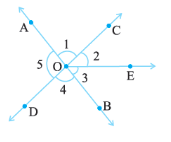Q

# In the adjoining figure : Is angle 1 adjacent to angle 2 ?

(iii) Do  and  form a linear pair?
(iv) Are  and  supplementary?
(v) Is  vertically opposite to  ?
(vi) What is the vertically opposite angle of  ?Views

(i) Yes,  adjacent to  as these have the same vertex and have one common arm.

(ii)  No,   is not adjacent to . This is because  contains .

(iii) Yes the given angles form a linear pair as they are pair of supplementary angles.

(iv) Since BOA is a straight line thus the given angles are supplementary.

(v) Yes,  and  are vertically opposite angles as they are the angles formed by two intersecting straight lines.

(vi) The vertically opposite angle to  is    .

Exams
Articles
Questions